### Solved Question Paper for Statistical Investigator Grade - I & Asstt. Director of Census Operations (T) in the Office of RGI conducted by UPSC

Solved Question Paper of Recruitment Test (Computer Based Recruitment Test) conducted by UPSC for the Post of Statistical Investigator Grade - I & Asstt. Director of Census Operations (T) in the Office of RGI held on 21.01.2017

1. The frequency distribution of wages is given below: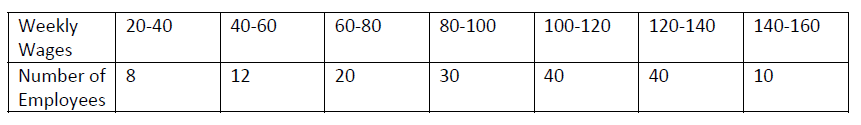The percentage of workers who earned between Rs 80 and Rs 125 is:
(a) 60%
(b) 50%
(c) 40%
(d) 30%

2. The following data are given on length (X) in cm and weight (Y) in gm of 6 tubes: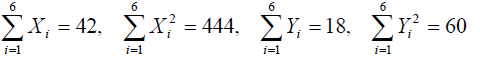Which one of the following is correct in respect of above data?
(a) X is more varying than Y
(b) Y is more varying than X
(c) X and Y have the same variance
(d) X and Y have the same mean

3. Let X , Y and Z denote the scores of golfers A, B and C respectively, in a match. The following data were obtained from 50 matches: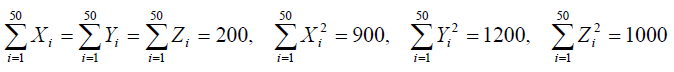The golfer who is most consistent is:
(a) A
(b) B
(c) C
(d) Cannot be determined

4.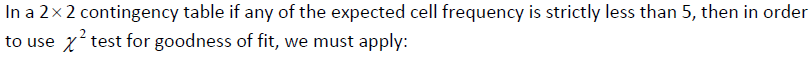(a) Gauss’s correction
(b) Sheppard’s correction
(c) Yates’ correction
(d) Bessel’s correction

5. Consider the following statements:
1. Karl Pearson’s correlation coefficient measures the degree of linear relationship between
two variables
2. Karl Pearson’s correlation coefficient lies between ‐1 and 1 including limits
3. Karl Pearson’s correlation coefficient is not independent of change of origin and scale

Which of the above statements is/ are correct?
(a) 1 and 2 only
(b) 1 only
(c) 2 and 3 only
(d) 1, 2 and 3

6. Consider the following statements:
1. The values of regression coefficients of Y on X and X on Y are 0.96 and 0.79 respectively.
2. Regression coefficients are independent of change of origin but not of scale.
3. Correlation coefficient is the geometric mean between regression coefficients.

Which of the above statements are correct?
(a) 2 and 3 only
(b) 1 and 3 only
(c) 1 and 2 only
(d) 1, 2 and 3

7. Consider the following statements:
1. Geometric mean is used to study the relative changes of price level in two time periods.
2. Geometric mean gives more weightage to small items compared to Harmonic mean.

Which of the above statements is/are correct?
(a) 1 only
(b) 2 only
(c) Both 1 and 2
(d) Neither 1 nor 2

8. Consider the following statements:
1. Quantitative data can be measured in ratio scale
2. Categorical data can be measured in either ordinal or interval scale.

Which of the above statements is/are correct?
(a) 1 only
(b) 2 only
(c) Both 1 and 2
(d) Neither 1 nor 2

9. Consider the following data about the classes A, B and C: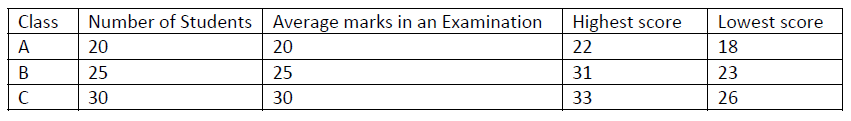If five students are transferred from A to B, then what will happen to the average score of B?
(a) It will remain constant
(b) It will definitely decrease
(c) It will definitely increase
(d) Cannot say

10. Consider the following statements:
1. Mean is not amenable to algebraic treatment.
2. Mode is not affected by extreme observations.
3. Median is not amenable to algebraic treatment.

Which of the above statements are correct?
(a) 1 and 2 only
(b) 1 and 3 only
(c) 2 and 3 only
(d) 1, 2 and 3

Share:

## Featured Post

### UPSC Civil Service Preliminary Paper-1 Previous Year Solved Question Papers

Civil Service Preliminary Paper-1 Previous Year Solved Questions for the year 2019 Civil Service Preliminary Paper-1 Previous Year Solved Qu...Name

Email *

Message *